# SIST Yang Wang’ s Research Group Publishes several academic papers at the IFAC WC2023 conference in automatic control field

Publisher：闻天明Release Time：2023-07-10Number of visits：10

Recently, three academic papers authored by postgraduate students Yizhou Gong, Yangyang Zhao, and Jiangkun Xu, from Professor Yang Wang’s research group in SIST, have been accepted at the International Federation of Automatic Control (IFAC) conference in the automatic control field.

Iteration Learning Control for Uncertain Nonlinear Systems with Time Varying Output Constraint

In this paper, a novel Adaptive Iteration Learning Control (AILC) method is proposed to solve the trajectory tracking problem for a class of nonlinear uncertain systems. The system in consideration has parametric uncertainties and is under the effect of external disturbances. Furthermore, the output of the system is required to be bounded by a time-varying function. To this end, a Barrier Lyapunov Function (BLF) term is integrated into the AILC scheme such that the impact of the uncertainties and disturbances are significantly reduced without violating the output constraints. A Barrier Composite Energy Function (BCEF) is utilized to analyze the convergence of state error and the boundedness of output. In addition, the validity of the proposed AILC scheme is verified by a numerical example. Finally, a high-fidelity simulation platform that can generate a real-life turbulent flow is utilized to demonstrate the robustness of the algorithm.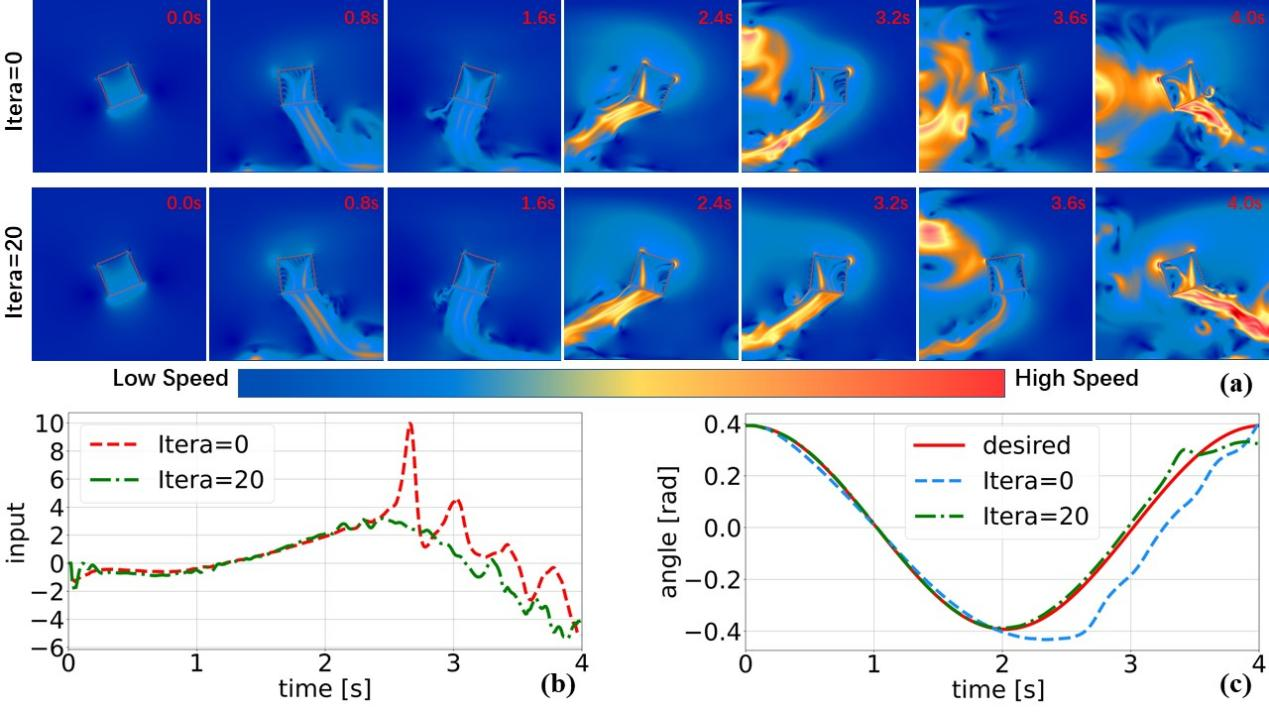Figure (a) fluid simulation schematic diagram, (b) control input iteration number, (c) tracking angle iteration number

The first author of this work is SIST master student Yangyang Zhao, and Dr. Yang Wang is the corresponding author.

Robust Output Regulation for Uncertain Linear Minimum Phase Systems under Unknown Control Direction

This paper investigates the problem of disturbance rejection for SISO uncertain linear minimum phase systems perturbed by an unmeasurable external disturbance under the framework of robust output regulation. The model parameters of systems in question are largely uncertain, including the control direction. In addition, the external disturbance is unstructured but bounded. Towards this end, a novel Unknown Input Observer (UIO)-based regulator is developed to reject the external disturbance, and a switching mechanism with a monitor function is designed to handle the control direction uncertainty. Notable features are that the unstructured external disturbance can be directly estimated and completely rejected by a sliding mode-based observer, and this new scheme can be applied for systems with non-unity relative degree under unknown control direction. The boundedness of closed-loop system and its asymptotic convergence properties are rigorously proved, which is verified by a numerical example.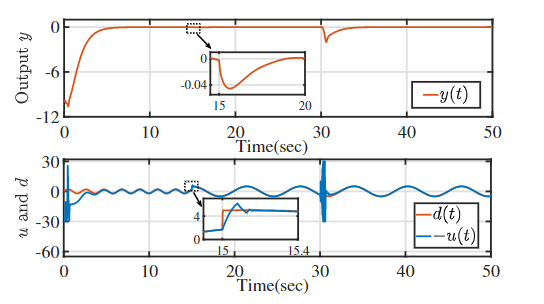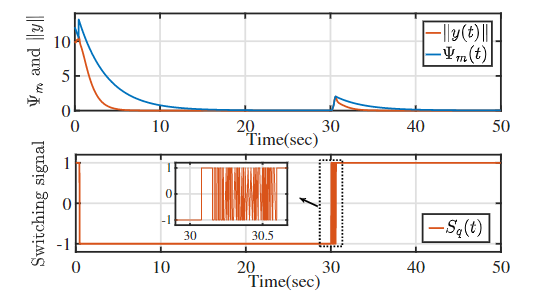Figure. 1. Trajectories of inputs and output of the system Figure. 2. Switching signal S and switching scheme with the monitoring function

The first author of this work is SIST master student Yizhou Gong, and Dr. Yang Wang is the corresponding author.

Robust Output Regulation for Uncertain Discrete-Time LTI Systems under the Effect of a Sinusoidal Disturbance

This paper studies the robust output regulation problem of uncertain single-input single-output (SISO) discrete-time linear systems. To reject the effect of a sinusoidal disturbance that only the frequency information is known prior, a novel strategy based on adaptive feedforward control (AFC) is developed. Compared with existing regulators for uncertain discrete-time systems, neither the knowledge on the sign of the real part or the imaginary part of the transfer function at the frequency of interest (the so-called SPR-like condition), nor persistence of excitation condition is required in this approach. Stability of the closed- loop system is rigorously analyzed using small-gain theorem and Lyapunov-based stability theory. Essentially, the proposed scheme guarantees that all signals of closed-loop system are bounded while the output of system asymptotically converges to zero, which is demonstrated by a numerical example.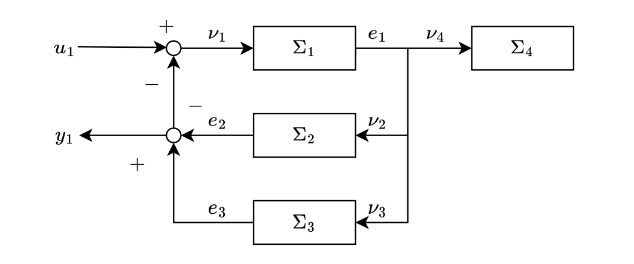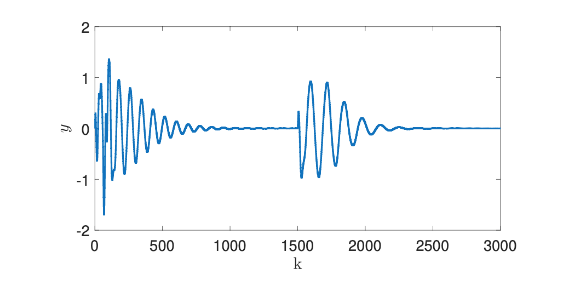Figure. 1. Interconnection of subsystems. Figure. 2. The regulated output of plant

The first author of this work is SIST master student Jiangkun Xu, and Dr. Yang Wang is the corresponding author.